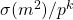# Some modular considerations regarding odd perfect numbers

Jose Arnaldo Bebita Dris and Immanuel Tobias San Diego
Notes on Number Theory and Discrete Mathematics
Print ISSN 1310–5132, Online ISSN 2367–8275
Volume 26, 2020, Number 2, Pages 27—33
DOI: 10.7546/nntdm.2020.26.2.27-33

## Details

### Authors and affiliations

Jose Arnaldo Bebita DrisDe La Salle University, Manila, Philippines 1004

Immanuel Tobias San DiegoDepartment of Mathematics and Physical Sciences
Trinity University of Asia, Quezon City, Philippines 1102

### Abstract

Letbe an odd perfect number with special prime. In this article, we provide an alternative proof for the biconditional thatholds if and only if. We then give an application of this result to the case whenis a square.

### Keywords

• Sum of divisors
• Sum of aliquot divisors
• Deficiency
• Odd perfect number
• Special prime

• 11A05
• 11A25

### References

1. Broughan, K. A., Delbourgo, D., & Zhou, Q. (2013). Improving the Chen and Chen result for odd perfect numbers, Integers, 13, Article #A39.
2. Chen, S.-C., & Luo, H. (2013). Odd multiperfect numbers, Bulletin of the Australian Mathematical Society, 88 (1), 56–63.
3. Ewell, J. A. Jr. (1980). On the multiplicative structure of odd perfect numbers, Journal of Number Theory, 12, 339–342.
4. Ochem, P. (2019). Answer to a question of the first author in Mathematics StackExchange, https://math.stackexchange.com/a/3151412/28816.
5. Sloane, N. J. A., OEIS sequence A033879 – Deficiency of n, or 2nσ(n), https://oeis.org/A033879.
6. Sloane, N. J. A., & Guy, R. K., OEIS sequence A001065 – Sum of proper divisors (or aliquot parts) of n: sum of divisors of n that are less than n, https://oeis.org/A001065.
7. Starni, P. (1991). On the Euler’s factor of an odd perfect number, Journal of Number Theory, 37, 366–369.
8. Wikipedia contributors. (2019, March 6). Perfect number. In Wikipedia, The Free Encyclopedia. Retrieved from https://en.wikipedia.org/w/index.php?title=Perfect_number&oldid=886493275.

## Cite this paper

Dris, J. A. B., & San Diego, I. T. (2020). Some modular considerations regarding odd perfect numbers, II. Notes on Number Theory and Discrete Mathematics, 26 (2), 27-33, doi: 10.7546/nntdm.2020.26.2.27-33.